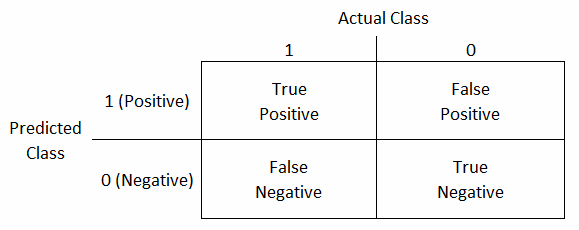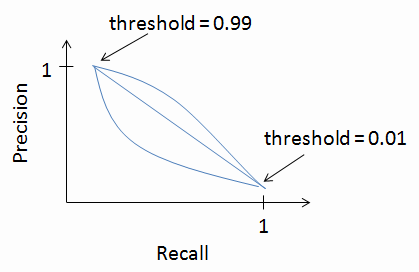### [學習筆記] 機器學習: 系統設計 (Machine Learning: System Design)

input (x): features of e-mail.

output (y): spam (y=1) or not spam (y=0).

1. 蒐集大量 training data

2. 建構精巧細緻的 features.

3. 開發演算法以不同的方式處理 inputs.

### 分析預測誤差 (error analysis)

1. 以簡單的演算法作為起點, 快速實作完成, 初期就以 cross validation data 測試演算法的準確度.

2. 繪製 learning curve, 用來判斷提升準確度的方向在於取得更多的 training data 或是納入更多的 features.

3. 檢視演算法對於 cross validation set 裡的 data 的預測誤差, 觀察大部分的誤差的原因是否有某種 trend, 據此設法降低誤差以提升準確度.

### 衡量 skewed classes 的指標 (Error metrics for skewed classes) - precision/recall

$$y = 1 \text{ if cancer}\\ y = 0 \text{ otherwise}$$$$\text{Accuracy} = \frac{\text{# of true positives + # of true negatives}}{\text{# of total examples}}$$

$$\text{Precision} = \frac{\text{# of true positives}}{\text{# of predicted positives}} = \frac{\text{# of true positives}}{\text{# of true positives + # of false positives}}$$

Precision 越高越好.
$$\text{Recall} = \frac{\text{# of true positives}}{\text{# of actual positives}} = \frac{\text{# of true positives}}{\text{# of true positives + # of false negatives}}$$

Recall 也是越高越好.
By convention, positive 通常設定為 $$y = 1$$, 且設定為發生機率較低的情況.

### Precision 和 recall 之間的取捨

Logistic regression: $$0 \leq h_{\theta}(x) \leq 1$$

Predict $$y=1$$ if $$h_{\theta}(x) \geq 0.5$$

Predict $$y=0$$ if $$h_{\theta}(x) \lt 0.5$$

Predict $$y=1$$ if $$h_{\theta}(x) \geq 0.7$$

Predict $$y=0$$ if $$h_{\theta}(x) \lt 0.7$$Predict $$y=1$$ if $$h_{\theta}(x) \geq 0.3$$

Predict $$y=0$$ if $$h_{\theta}(x) \lt 0.3$$Predict $$y=1$$ if $$h_{\theta}(x) \geq \text{threshold}$$Algorithm 1: P = 0.4, R = 0.5 → Average = 0.45

Algorithm 2: P = 0.01, R = 1.0 (例如 always predict y = 1) → Average = 0.505

$$F_1 \text{Score} = 2 \frac{P * R}{P + R}$$

### Training data 數量的考量1. Features $$x \in \mathbb{R}^{n+1}$$ 有足夠的資訊來準確地預測 y.

2. 採用具有許多 parameters 的 learning algorithm.

# 系列文章

[學習筆記] 機器學習: 簡介 (Machine learning: Introduction)

[學習筆記] 機器學習: 單變數線性回歸 (Machine learning: Linear Regression with One Variable)

[學習筆記] 機器學習: 多變數線性回歸 (Machine learning: Linear Regression with Multiple Variables)

[學習筆記] 機器學習: 邏輯回歸 (Machine learning: Logistic Regression)

[學習筆記] 機器學習: 正規化 (Machine learning: Regularization)

[學習筆記] 機器學習: 神經網路的結構 (Machine Learning: Neural Networks: Representation)

[學習筆記] 機器學習: 神經網路的學習 (Machine Learning: Neural Networks: Learning)

[學習筆記] 機器學習: 應用上的建議 (Machine Learning: Advice for Applying Machine Learning)

[學習筆記] 機器學習: 系統設計 (Machine Learning: System Design)

[學習筆記] 機器學習: 支援向量機 (Machine Learning: Support Vector Machines)

[學習筆記] 機器學習: 非監督式學習 (Machine Learning: Unsupervised Learning)

[學習筆記] 機器學習: 維度縮減 (Machine Learning: Dimensionality Reduction)

[學習筆記] 機器學習: 異常偵測 (Machine Learning: Anomaly Detection)

[學習筆記] 機器學習: 推薦系統 (Machine Learning: Recommender Systems)

[學習筆記] 機器學習: 大量資料機器學習 (Machine Learning: Large Scale Machine Learning)

# 延伸閱讀

[Coursera] Machine Learning: Machine Learning System Design, by Andrew Ng, Stanford University